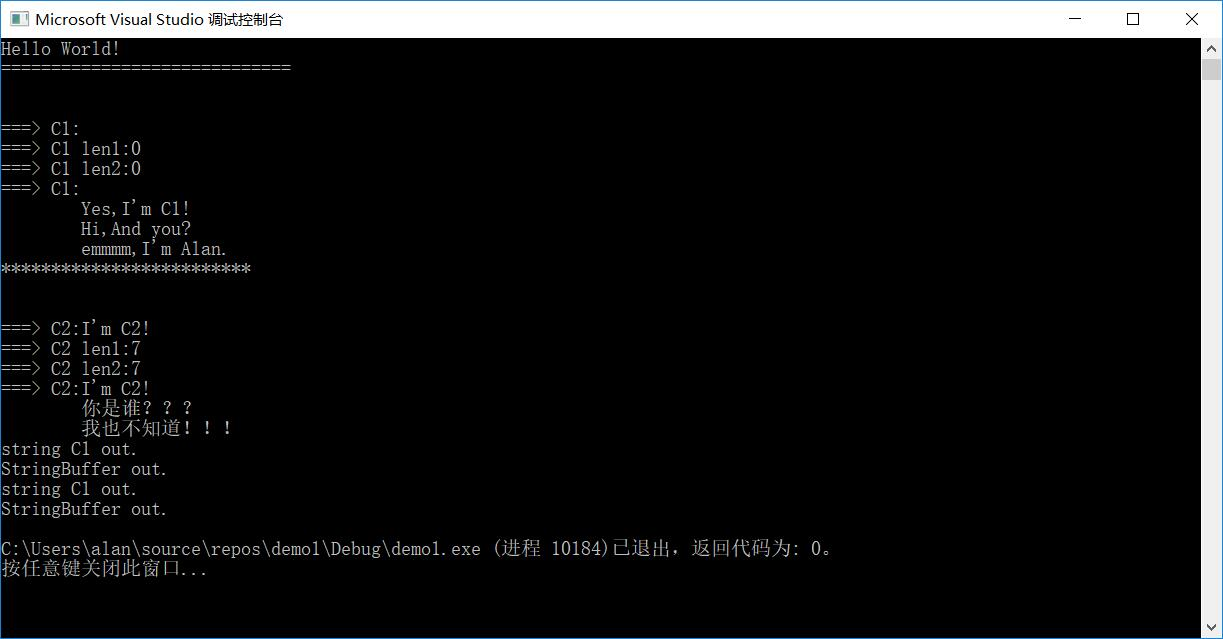## HTTP状态码（记录）

HTTP状态码总的分为五类：

1开头：信息状态码

2开头：成功状态码

3开头：重定向状态码

4开头：客户端错误状态码

5开头：服务端错误状态码

1XX：信息状态码

 状态码 含义 描述 100 继续 初始的请求已经接受，请客户端继续发送剩余部分 101 切换协议 请求这要求服务器切换协议，服务器已确定切换

2XX：成功状态码

 状态码 含义 描述 200 成功 服务器已成功处理了请求 201 已创建 请求成功并且服务器创建了新的资源 202 已接受 服务器已接受请求，但尚未处理 203 非授权信息 服务器已成功处理请求，但返回的信息可能来自另一个来源 204 无内容 服务器成功处理了请求，但没有返回任何内容 205 重置内容 服务器处理成功，用户终端应重置文档视图 206 部分内容 服务器成功处理了部分GET请求

3XX：重定向状态码

 状态码 含义 描述 300 多种选择 针对请求，服务器可执行多种操作 301 永久移动 请求的页面已永久跳转到新的url 302 临时移动 服务器目前从不同位置的网页响应请求，但请求仍继续使用原有位置来进行以后的请求 303 查看其他位置 请求者应当对不同的位置使用单独的GET请求来检索响应时，服务器返回此代码 304 未修改 自从上次请求后，请求的网页未修改过 305 使用代理 请求者只能使用代理访问请求的网页 307 临时重定向 服务器目前从不同位置的网页响应请求，但请求者应继续使用原有位置来进行以后的请求

4XX：客户端错误状态码

 状态码 含义 描述 400 错误请求 服务器不理解请求的语法 401 未授权 请求要求用户的身份演验证 403 禁止 服务器拒绝请求 404 未找到 服务器找不到请求的页面 405 方法禁用 禁用请求中指定的方法 406 不接受 无法使用请求的内容特性响应请求的页面 407 需要代理授权 请求需要代理的身份认证 408 请求超时 服务器等候请求时发生超时 409 冲突 服务器在完成请求时发生冲突 410 已删除 客户端请求的资源已经不存在 411 需要有效长度 服务器不接受不含有效长度表头字段的请求 412 未满足前提条件 服务器未满足请求者在请求中设置的其中一个前提条件 413 请求实体过大 由于请求实体过大，服务器无法处理，因此拒绝请求 414 请求url过长 请求的url过长，服务器无法处理 415 不支持格式 服务器无法处理请求中附带媒体格式 416 范围无效 客户端请求的范围无效 417 未满足期望 服务器无法满足请求表头字段要求

5XX：服务端错误状态码

 状态码 含义 描述 500 服务器错误 服务器内部错误，无法完成请求 501 尚未实施 服务器不具备完成请求的功能 502 错误网关 服务器作为网关或代理出现错误 503 服务不可用 服务器目前无法使用 504 网关超时 网关或代理服务器，未及时获取请求 505 不支持版本 服务器不支持请求中使用的HTTP协议版本

## Windows下JetBrains CLion中文输出乱码的解决方法

JetBrains公司推出的 C/C++ IDE CLion，在编写代码时效率非常高，相信以后也会成为开发C/C++项目的首选。

CLion输出中文时，都会产生乱码，不过也有解决办法，示例代码如下：

#include <iostream>

using namespace std;

int main() {
std::cout << “Hello, World!” << std::endl;
cout << “速度嘎是的反垃圾开始都看过是对方感觉啊” << endl;
return 0;
}

Hello, World!

Process finished with exit code 0

File->Setting->Editor->File Encodings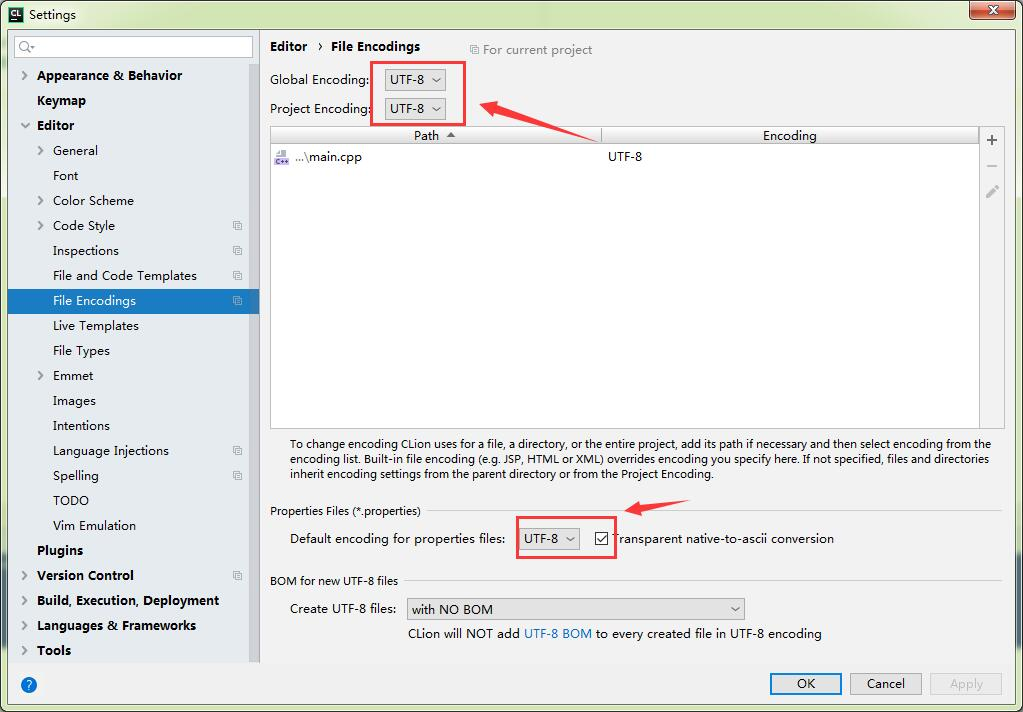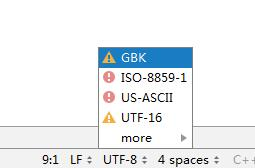## Java语言Volatile原理

Java语言提供了一种稍弱的同步机制，即volatile变量，用来确保将变量的更新操作通知到其他线程。当把变量声明为volatile类型后，编译器与运行时都会注意到这个变量是共享的，因此不会将该变量上的操作与其他内存操作一起重排序。volatile变量不会被缓存在寄存器或者对其他处理器不可见的地方，因此在读取volatile类型的变量时总会返回最新写入的值。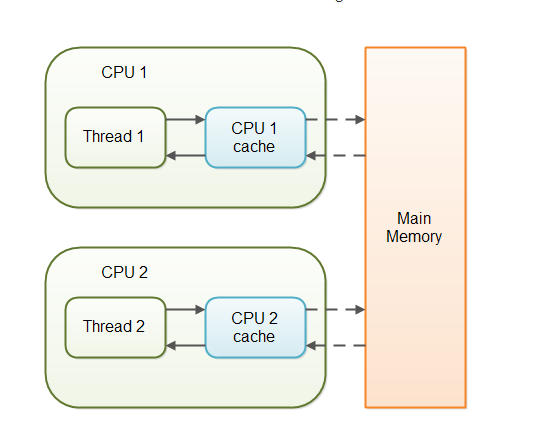### 当一个变量定义为 volatile 之后，将具备两种特性：

1.保证此变量对所有的线程的可见性，这里的“可见性”，当一个线程修改了这个变量的值，volatile 保证了新值能立即同步到主内存，以及每次使用前立即从主内存刷新。但普通变量做不到这点，普通变量的值在线程间传递均需要通过主内存来完成。

### volatile 性能：

volatile 的读性能消耗与普通变量几乎相同，但是写操作稍慢，因为它需要在本地代码中插入许多内存屏障指令来保证处理器不发生乱序执行。

## 快排算法的实现与讲解(java/C++)

6 >= 5 条件成立，接着左边往右边移动一位（j–）

……

4>=5条件不成立，这个时候就换一下位置，4跟5换。现在的数组应该就是这样子：{4,2,1,8,9,3,7,0,5,6}

5>=4条件成立，右边往左边靠拢（i++）

5>=2条件成立，右边往左边靠拢（i++）

……

5>=8条件不成立，换位置：{4,2,1,5,9,3,7,0,8,6}

{4,2,1,0,9,3,7,5,8,6}

{4,2,1,0,5,3,7,9,8,6}

….以此类推，哨兵i继续向右移动，悲剧！！！此时i和j撞上了，说明此时“探测”结束。我们将基准数5和3进行交换。交换之后的序列如下：

{4,2,1,0,3,5,7,9,8,6}

{4,2,1,0,3,        5       ,7,9,8,6}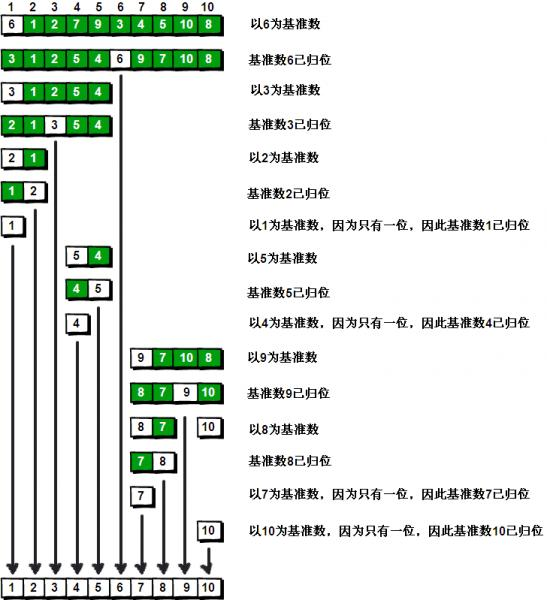• 快排的原理很简单；
• 就是把数组分为两节；
• 左边的是最小的，而右边的是最大的；
• 然后再拿左、右边的来继续递归，递归的原理也一样，也是拆分为两节，以此类推。

2.java代码:

```public static void main(String[] args) {
int[] arr = {5,2,1,8,9,3,7,0,4,6};
sort(arr, 0, arr.length - 1);
for (int i : arr) {
System.out.print(i + " ");
}
}

private static void sort(int[] arr, int l, int r) {
int i = l;
int j = r;
if (l < r) {
while (l < r) {
while (l < r && arr[r] >= arr[l]) {
r--;
}
int tmp = arr[l];
arr[l] = arr[r];
arr[r] = tmp;

while (l < r && arr[l] <= arr[r]) {
l++;
}
tmp = arr[l];
arr[l] = arr[r];
arr[r] = tmp;

}
sort(arr, i, l - 1);//递归左边，此时l=5
sort(arr, l + 1, j);//递归右边，此时l=5
}
}```

2.那么c++的代码会是怎么样呢？

```#include "pch.h"
#include <iostream>

using namespace std;

void sortQ(int *arr, int l, int r) {
int i = l;
int j = r;
int tmp;
if (i < j) {
while (l < r) {
while (l < r && arr[r] >= arr[l])
{
r--;
}

tmp = arr[l];
arr[l] = arr[r];
arr[r] = tmp;

while (l < r && arr[r] >= arr[l]) {
l++;
}
tmp = arr[l];
arr[l] = arr[r];
arr[r] = tmp;
}

sortQ(arr, i, l - 1);//处理左边，此时l=5
sortQ(arr, l + 1, j);//处理右边，此时l=5
}

}

int main()
{
std::cout << "Hello World!\n";

int arr[] = {5,2,1,8,9,3,7,0,4,6};
int size = sizeof(arr) / sizeof(arr);

sortQ(arr, 0, size - 1);
for (int i : arr) {
cout << i << " ";
}

}```

## 使用C/C++新建了一个类似于Java的StringBuffer类

1.StringBuffer & append(const char * _c) ;

2.StringBuffer(const StringBuffer & buf);

3.char* toString();

4.int length();

5.std::string substr(int start = 0,int len = 1);

6.void replaceAll(const char * find, const char * des);

7.int indexOf(const char * find);

8.bool operator==(const StringBuffer & buf);
C/C++中特有的方法，如果需要使用运算符：a==b，增需要定义一个自定义的运算符方法，这里只定义了：==。

```#pragma once
#include<iostream>

//exmple:
/*
// StringBuffer sb;
// or like this:
// StringBuffer sb("test");
// sb.append("append.");

// and you can like this:
// sb.append("append 1,").append("append 2,");

// equal:
// sb1==sb?1:0
// if(sb1 == sb){//do something...}

// length:
// sb.length();

// substring:
// sb.substr(int strat,int end);
// sb.substr(0,1);//it will be return "t";

// find:
// sb.indexOf(char * find_char);
// sb.indexOf("st");//it will be return 2;
// sb.indexOf("index");//it will be return -1;

// replaceAll:
// sb.replaceAll(char * find_char,char * des_char);
// like this:
// sb.replaceAll("test","1234");

// toString:
// sb.toString();
*/

#ifndef STRING_BUFFER
#define STRING_BUFFER

class StringBuffer
{
private:
char * value;
char * value1;
int len;
int _number;
public:
StringBuffer();
StringBuffer(const char * _c);
StringBuffer & append(const char * _c) ;
StringBuffer(const StringBuffer & buf);
char* toString();
int length();
std::string substr(int start = 0,int len = 1);
void replaceAll(const char * find, const char * des);
int indexOf(const char * find);
bool operator==(const StringBuffer & buf);
~StringBuffer();
};

#endif // !STRING_BUFFER

```

```#include "pch.h"
#include "StringBuffer.h"
#include<iostream>
#include<string.h>

StringBuffer::StringBuffer()
{
len = 1;
_number = 0;
value = new char{"\0"};
}

StringBuffer::StringBuffer(const char * _c) {
len = std::strlen(_c) + 1;
_number = 1;
value = new char[len];
strcpy_s(value, len, _c);
}

StringBuffer::StringBuffer(const StringBuffer & buf) {
len = buf.len;
_number = buf._number;
value = new char[len];
strcpy_s(value, len, buf.value);
}

StringBuffer::~StringBuffer()
{
delete[] value;
std::cout << "StringBuffer out." << std::endl;
}

StringBuffer & StringBuffer::append(const char * _c) {
int _l = std::strlen(_c)+1;
value1 = new char[len+_l];
strcpy_s(value1, len, value);
strcat_s(value1, len + _l, _c);

value = value1;

len += _l;
_number++;
return *this;
}

char* StringBuffer::toString() {
return this->value;
}

int StringBuffer::length() {
return strlen(value);
}

std::string StringBuffer::substr(int start, int len) {
std::string s = value;
return s.substr(start, len);
}

int StringBuffer::indexOf(const char * find) {
std::string s = value;
return s.find(find);
}

void StringBuffer::replaceAll(const char * find, const char * des) {
int idx = 0;
int _len = std::strlen(find);
bool has = false;
std::string s = value;
while (idx > -1)
{
idx = s.find(find);
if (idx > -1) {
s = s.replace(idx, _len, des);
has = true;
}
}

if (has) {
strcpy_s(value, strlen(s.data()) + 1, s.data());
len = strlen(s.data()) + 1;
value1 = value;
}

}

bool StringBuffer::operator==(const StringBuffer & buf) {
if (this == &buf) {
return true;
}
return strcmp(this->value, buf.value) == 0;
}```